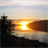QlikView App Dev

Discussion Board for collaboration related to QlikView App Development.

Announcements
QlikWorld 2022, LIVE in Denver CO., May 16-19, 2022. REGISTER NOW TO RECEIVE EARLY BIRD PRICING
cancel
Showing results for
Did you mean:Creator

max value of date in possible selections of date

Hi All,

I have a field called Period(DD/MM/YYYY) which is basically my Calendar Date field. There is another field called R_Period (DD/MM/YYYY) which is like past 12 months to every value in Period field. Now I need to show this in chart i.e. for every Period(Period as dimension), Sum of Quantity of only the max R_Period's month.

I have to do it using set analysis.

I tried:

(SUM({<R_Period = {"=Aggr(max(Period),Month)"}>}Retail_Qty))

also,

sum({<R_Period={'\$(=max(R_Period))'}>}Retail_Qty)

also,

sum(aggr(if(rank(R_Period)=1,sum(Retail_Qty)),R_Period))

Regards,

Ruchi

3 RepliesChampion II

Can you post expected output as well, what exactly are you trying to acheive

if you just want total for max , then your second expression will work

sum({<R_Period = {'\$(=MAX(R_Period))'}>}Retail_Qty)Creator
Author

Hi Vineeth,

For each Period ( Jul 2016, Aug 2016, Sep 2016, Oct 2016...) it has a set of its own 12 months data(R_Period) eg:

Jul 2016 (Period ) has (Aug 2015 to Jul 2016)(R_Period).

Expected output :

For each Period, it calculates the maximum of R_Period and sums the Quantity on it. Whereas what this expression does is, it does calculate the max of R_Period but shows only for max Period. Hence In my chart I get bar only for the max Period (Oct 2016), I want to see for all the Periods.

Regards,

RuchiChampion II

Can you create an expected output table in excel, using the sample you posted for any period.Community Browser